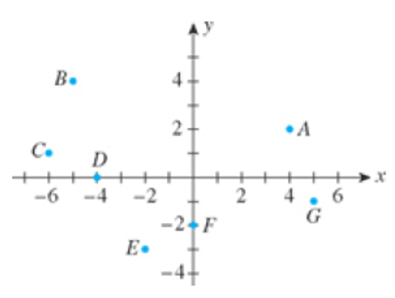Chapter 1.3, Problem 9E

Chapter
Section
Textbook Problem

In Exercises 7-12, refer to the following figure.9. Which points have negative y-coordinates?

To determine
The point which have negative y-coordinate.

Explanation

Given:

The figure is,

Figure (1)

Calculation:

The xy-plane is divided into 4 quadrants. Quadrant I consists of the points P(x,y) that satisfy x>0 and y>0 ; Quadrant II consists of the points P(x,y) , where x<0 and y>

Still sussing out bartleby?

Check out a sample textbook solution.

See a sample solution

The Solution to Your Study Problems

Bartleby provides explanations to thousands of textbook problems written by our experts, many with advanced degrees!

Get Started

Prove the statement using the , definition of a limit. limx6+6+x8=0

Single Variable Calculus: Early Transcendentals, Volume I

Sum of Square (SS), variance and standard deviation

Statistics for The Behavioral Sciences (MindTap Course List)

In problems 63-73, factor each expression completely. 64.

Mathematical Applications for the Management, Life, and Social Sciences